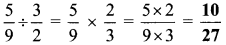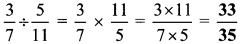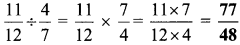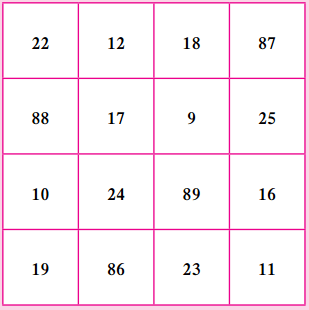# Maharashtra Board Practice Set 13 Class 6 Maths Solutions Chapter 4 Operations on Fractions

Balbharti Maharashtra State Board Class 6 Maths Solutions covers the Std 6 Maths Chapter 4 Operations on Fractions Class 6 Practice Set 13 Answers Solutions.

## 6th Standard Maths Practice Set 13 Answers Chapter 4 Operations on Fractions

Question 1.
Write the reciprocals of the following numbers:

1. 7
2. $$\frac { 11 }{ 3 }$$
3. $$\frac { 5 }{ 13 }$$
4. 2
5. $$\frac { 6 }{ 7 }$$

Solution:

1. $$\frac { 1 }{ 7 }$$
2. $$\frac { 3 }{ 11 }$$
3. $$\frac { 13 }{ 5 }$$
4. $$\frac { 1 }{ 2 }$$
5. $$\frac { 7 }{ 6 }$$

Question 2.
Carry out the following Divisions:
i. $$\frac{2}{3} \div \frac{1}{4}$$
ii. $$\frac{5}{9} \div \frac{3}{2}$$
iii. $$\frac{3}{7} \div \frac{5}{11}$$
iv. $$\frac{11}{12} \div \frac{4}{7}$$
Solution:
i. $$\frac{2}{3} \div \frac{1}{4}$$ii. $$\frac{5}{9} \div \frac{3}{2}$$iii. $$\frac{3}{7} \div \frac{5}{11}$$iv. $$\frac{11}{12} \div \frac{4}{7}$$Question 3.
There were 420 students participating in the Swachh Bharat Campaign. They cleaned $$\frac { 42 }{ 75 }$$ part of the town, Sevagram. What part of Sevagram did each student clean if the work was equally shared by all?
Solution:
Total number of students = 420
Part of town cleaned by all the students = $$\frac { 42 }{ 75 }$$
∴ Part of town cleaned by one student∴ Part of town cleaned bv one student is $$\frac { 1 }{ 750 }$$

#### Maharashtra Board Class 6 Maths Chapter 4 Operations on Fractions Practice Set 13 Intext Questions and Activities

Question 1.
Ramanujan’s Magic square. (Textbook pg. no. 28)• Add the four numbers in the rows, the columns and along the diagonals of this square.
• What is the sum?
• Is it the same every time?
• What is the peculiarity?
• Look at the numbers in the first row, 22 – 12 – 1887. Find out why this date is special.

Obtain and read a biography of the great Indian mathematician Srinivasa Ramanujan.
Solution:
Sum of the numbers in each row:
i. 22 + 12 + 18 + 87 = 139
ii. 88 + 17 + 9 + 25 = 139
iii. 10 + 24 + 89 + 16 = 139
iv. 19 + 86 + 23 + 11 = 139

Sum of the numbers along the diagonals:
i. 22 + 17 + 89 + 11 = 139
ii. 87 + 9 + 24 + 19 = 139

Sum of the numbers in each column:
i. 22 + 88 + 10 + 19 = 139
ii. 12 + 17 + 24 + 86 = 139
iii. 18 + 9 + 89 + 23 = 139
iv. 87 + 25 + 16 + 11 = 139

∴ We observe that the sum of the numbers in each of the rows, the columns and along each diagonal remains the same every time. The numbers in the first row 22 – 12 – 1887 is the birth date of Srinivasa Ramanujan.